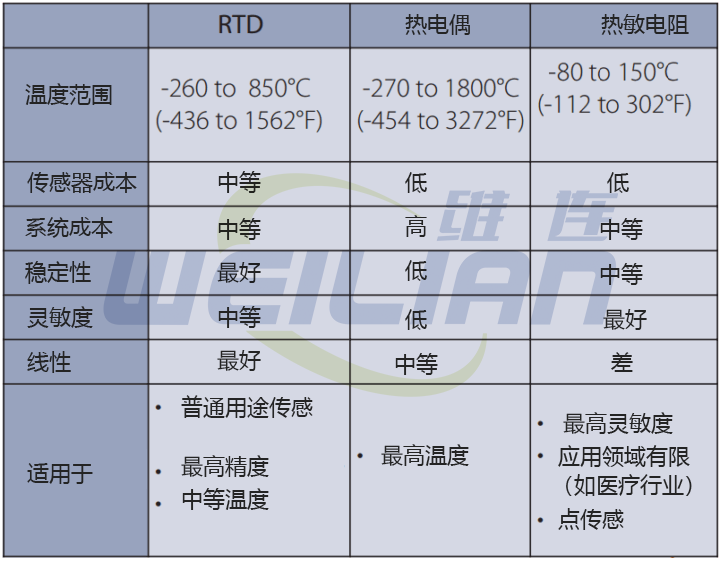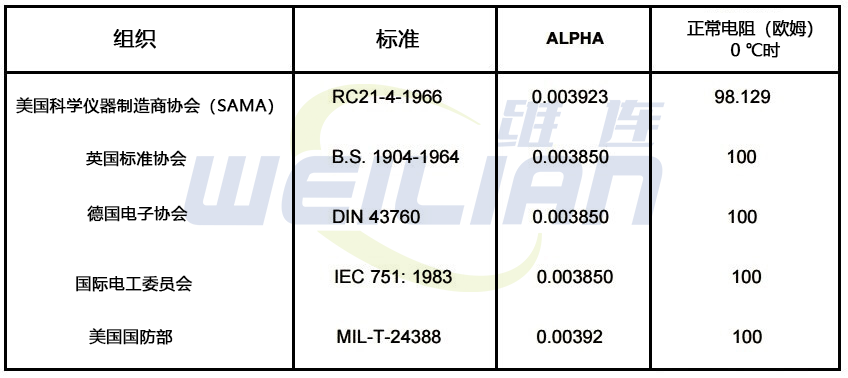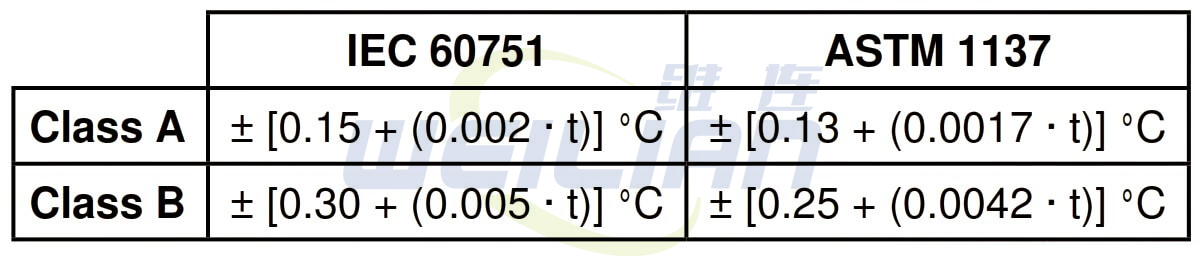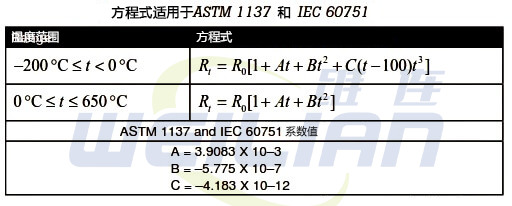### 如何计算 RTD温度传感器的公差

2021-09-08       来源：www.weilianchina.com如何计算 RTD 传感器的公差DIN/IEC751:2008 中实际上规定了四种电阻公差

AA 级 = ± ( 0.10 + 0.0017 * | t | ) °C

A 级 = ± ( 0.15 + 0.0020 * | t | ) °C

B 级 = ± ( 0.30 + 0.0050 * | t | ) °C

C 级 = ± ( 0.60 + 0.0100 * | t | ) °CA 级 = ± [0.13 + (0.0017 · t)] °C
= (0.13 + (0.0017 · t)) · 0.1
= (0.13 + (0.0017 · 100)) · 0.1
= (0.13 + 0.17) · 0.1
= 0.0.0维连在线客服
>
Top